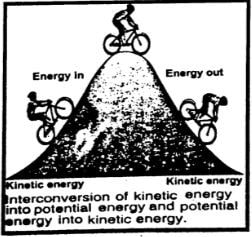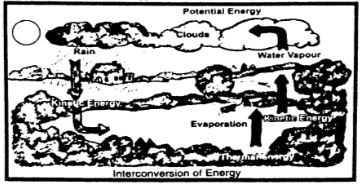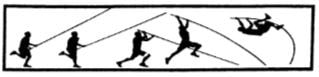Define work. What is its SI unit?

Difficulty: Medium

Work:

Work is done when a force acting on a body displaces it in the direction of a force. Work is a scalar quantity. It depends on the force acting on a body, displacement of the body, and the angle between them.

Work done = Force x displacement

Or          W = F S........ (i)

W = $F_{x} \times S$
W = $(F cos \theta) S$
W = $FS cos \theta$ ........(ii)

Unit of work:

SI unit of work is the joule (J). it is defined as

The amount of work is one joule when a force of one newton displaces a body through one meter in the direction of the force.

Thus                              $1 J = 1 N \times 1 m$

When does a force do work? Explain.

Difficulty: Medium

Work is said to be done when a force acts on a body and moves it in the direction of the force. Greater is the force acting on a body and the the the longer is the distance moved by it, the the the larger would be the work done. Mathematically, Work is a product of force F and displacement S in the direction of the force. Thus

Work done = Force x displacement

Or             W = F S .........(i)

If the force is unbalanced, it causes acceleration. This involves doing work to move it in a certain direction.

Why do we need energy?

Difficulty: Medium

Need for energy:

Energy is a fundamental concept in science. It links almost all the natural phenomena. When we say that a body has energy, we mean that it can do work, so it possesses energy. The energy from running water can be used to run the water mills or water turbines.

Energy exists in various forms such as mechanical energy, heat energy, light energy, sound energy, electrical energy, chemical energy, nuclear energy, etc. Energy can be transformed from one form into another.

Define energy, give two types of mechanical energy.

Difficulty: Easy

Energy:

A body possesses energy if it is capable to do work.

Types of mechanical energy:

Mechanical energy possessed by a body is of two types: kinetic energy and potential energy.

Define K.E. and derive its relation.

OR

Prove that K.E. =   K.E. =  $\frac{1}{2} m v^{2}$

Difficulty: Medium

Kinetic Energy:

The energy possessed by a body due to its motion is called its kinetic energy.

Derivation of K.E:

Consider a body of mass m moving with velocity v. The body stops after moving through some distance S due to some opposing force such as the force of friction acting on it. The body possesses kinetic energy and is capable to do work against opposing force F until all of its kinetic energy is used up.

K.E. of the body = Work done by it due to motion

K.E      =        FS.......... (i)

Vi         =        v

Vf        =        0

As                                              F =  ma

A   =   $-\frac{F}{m}$

Since motion is opposed, hence, a is negative. Using 3rd equation of motion:

$2aS=V_f^2- V_i^2$

$2 (- F/m) S = (0)2 – (v)2$

F S =$\frac{1}{2} m v^{2}$........(ii)

From Eq. (i) and (ii), we get

K.E. =  $\frac{1}{2} mv^{2}$.......(iii)

Equation (iii) gives the K.E. possessed by a body of mass m moving with velocity v.

Define potential energy and derive its relation?

Difficulty: Easy

Potential Energy:

The energy possessed by a body due to its position is known as its potential energy.

Derivation of P.E:

Let a body of mass m be raised through height h from the ground. The body will acquire potential energy equal to the work done in lifting it to height h.

Thus, Potential energy  P.E. =$F\times h$
= $w\times h$

(Here weight of the body = w = mg)

P.E.= w h= mgh………(i)

Thus, the potential energy possessed by the body concerning the ground is much and is equal to the work done in lifting it to height h.

Why are fossil fuels called a non-renewable form of energy?

Difficulty: Easy

Fossil fuels are nonrenewable resources because they took millions of years to attain their present form.

We use fossil fuels such as coal, oil, and gas to heat our houses, run industry, and transport. They are usually hydrocarbons (compounds of carbon and hydrogen). When they are burnt, they combine with oxygen from the air. The carbon becomes carbon dioxide; hydrogen becomes hydrogen oxide called water; while energy is released as heat. In the case of coal:

Carbon + Oxygen      $\rightarrow$  carbon dioxide + heat energy

Hydrocarbon + Oxygen    $\rightarrow$   carbon dioxide + water + heat energy

Which form of energy is most preferred and why?

Difficulty: Easy

Solar energy is the most preferred.

Explanation:

Solar energy is the energy coming from the Sun and is used directly and indirectly. Sunlight does not pollute the environment in any way. The sunrays are the ultimate source of life on Earth. We are dependent on the Sun for all our food and fuels. If we find a suitable method to use a fraction of the solar energy reaching the Earth, then it would be enough to fulfill our energy requirement.

How is energy converted from one form to another? Explain.

Difficulty: Medium

Interconversion of energy:

Energy cannot be destroyed however it can be converted into some other forms.

Examples:

1. Rub your hands together quickly. You will feel them warm. You have used your muscular energy in rubbing hands as a result heat is produced. In the process of rubbing hands, mechanical energy is converted into heat energy.2. Processes in nature are the results of energy changes. For example, some of the heat energy from the Sun is taken up by the water in the oceans. This increases the thermal energy.Thermal energy causes water to evaporate from the surface to form water vapors. These vapors rise and form clouds. As they cool down, they form water drops and fall as rain. Potential energy changes to kinetic energy as the rain falls, this rainwater may reach a lake or a dam. As the rainwater flows down, its kinetic energy changes into thermal energy while parts of the kinetic energy of flowing water are used to wash away soil particles of rocks known as soil erosion.

Note: During the interconversion of energy from one form to other forms, the total energy at any time remains constant.

DO YOU KNOW?Pole vaulter:

A pole vaulter uses a flexible vaulting pole made of special material. It is capable to store all the vaulter's kinetic energy while bending in the form of potential energy. The vaulter runs as fast as possible to gain speed. The kinetic energy gained by the pole vaulter due to speed helps him/her to rise as the vaulter straightens. Thus, he attains height as the pole returns the potential energy stored by the vaulter in the pole.

Name five devices that convert electrical energy into mechanical energy.

Difficulty: Easy

(i)     Electric motor converts electrical energy to mechanical energy.

(ii)    Drill machine

(iii)    Electric fan

(iv)   Electric spinner

(v)    Electric grinder

Name a device that converts mechanical energy into electrical energy.

Difficulty: Easy

An electric generator converts mechanical energy into electrical energy.

What is meant by the efficiency of a system?

OR

How can you find the efficiency of a system?

Difficulty: Medium

Efficiency:

The ratio of the useful work done by a device or machine to the total energy taken up by it is called its efficiency.

OR

The efficiency of a system is the ratio of the required form of energy obtained from a system as output to the total energy given to it as input.

Efficiency= $\frac{(required form of output)}{(total input energy)}$..... (i)

% Efficiency= $\frac{ (required form of output)}{(total input energy)}×100$ ......(ii)

Ideal system/Ideal machine:

An ideal system is that which gives an output equal to the total energy used by it. In other words, its efficiency is 100 %.

People have tried to design a working system that would be 100 % efficient. But practically such a system does not exist.

 Efficiencies of some typical devices/machines Energy Input Device or machines Useful Work done % Efficiency 100 J Electric lamp 5 J 5 % 100 J Patrol Engine 25 J 25 % 100 J Electric Motor 80 J 80 % 100 J Electric Fan 55 J 55 % 100 J Solar Cell 3 J 3 %

What is meant by the term power? Define watt.

Difficulty: Medium

Power is defined as the ratio of doing work.

Mathematically,

Power                 P= $\frac{Work \: done}{Time \: taken}$

Or                      P= $\frac{W}{t}$………… (i)

Since work is a scalar quantity, therefore, power is also a scalar quantity.

Note:     Bigger units of power are kilowatt (kW), megawatt (MW), etc.

1 kW=1000W=10^{3}

1MW=1000 000=10^{6}

1 horsepower= 1hp = 746 W

Define watt.

Difficulty: Easy

Unit of power

SI unit of power is the watt (W). It is defined as

Watt:

The power of a body is one watt if it does work at the rate of 1 joule per second $(1 J s ^{-1})$